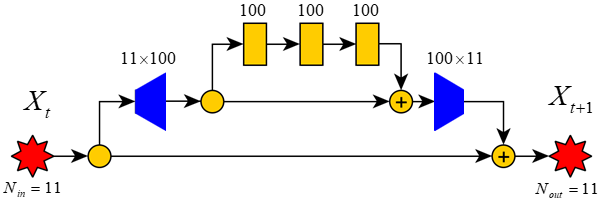Seminar “Simulation of hydrogen combustion dynamics using a fully connected UNET neural network”

# Seminar “Simulation of hydrogen combustion dynamics using a fully connected UNET neural network”

The event passed
9 Sep 2021
Location
Online

September 9 at 16:30 MSK

Calculation of physicochemical interactions of gas-dynamic processes taking place in engines is a laborious task. If a non-stationary process is calculated, including such physical phenomena as detonation, ignition of a combustible mixture, propagation of combustion waves, then obtaining calculated data even for a very short physical time requires a long operation of supercomputer systems. An attempt to speed up the calculation time using the usual simple methods, such as coarsening of the computational grid or extrapolation of the results obtained at the initial stage of the calculation for a much longer time period, does not guarantee the absence of gross deviations of the numerical from the original mathematical model. In this work, we consider the problem of combustion of hydrogen with oxygen in the presence of neutral elements (nitrogen (N2) and argon (Ar)). During combustion, various hydrogen-oxygen compounds are formed (H2, O2, H2O, OH, HO2, H2O2, H, O), the temperature of the mixture changes. This process of transformation of substances is described by 28 equations of chemical reactions (mechanism GriMech 3.0 (1999)).

As a result, it is required to trace the development of this system from some initial state by several steps in time. This paper shows that the UNET architecture neural network can be successfully applied to the problem of predicting deterministic multidimensional time series, namely, modeling the chemical combustion processes described by a rigid system of ordinary differential equations. Using it, we were able to train a compact model that can approximate changes in the concentrations of substances in a mixture during chemical reactions with a high degree of accuracy, sufficient to recursively obtain predictions for hundreds and even thousands of integration steps forward, while taking an order of magnitude less computation time than numerical integration. This work is a development of the ideas described in work . By changing the data normalization and the network architecture (see figure), we managed not only to improve the accuracy and reliability of the neural network, but also to reduce computational costs.

 V.B. Betelin, B.V. Kryzhanovsky, N.N. Smirnov, V.F. Nikitin, I.M. Karandashev, M. Yu Malsagov, E.V. Mikhalchenko, Neural network approach to solve gas dynamics problems with chemical transformations, Acta Astronautica, 2020, ISSN 0094-5765, https://doi.org/10.1016/j.actaastro.2020.11.058Figure. UNET neural network architecture

### Speaker

Iakov Karandashev, Candidate of Physical and Mathematical Sciences, Leading Researcher of SRISA RAS, Associate Professor of S.M. Nikolsky Mathematical Institute of RUDN

Related eventsAll events
2023
4 Oct
Seminar “Environmental Protection in Agriculture”
2023
28 Sep
Seminar “Dynamics of Persistent Epidemic and Optimal Control of Vaccination”
2023
5 Oct
Lecture “Metal Catalysis in Chemoselective Peptide Macrocyclization”
2023
4 Oct
Lecture “Multicomponent Synthesis of Macrocyclic and Stapled Peptides”